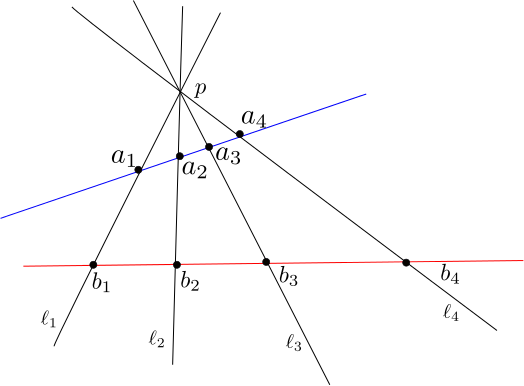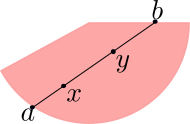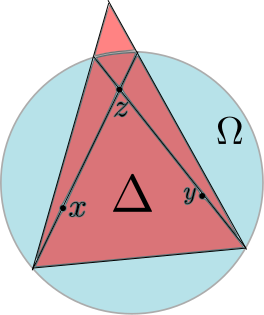In this lecture, we will discuss the geometry of properly convex subsets of $$\mathbb{R} P^n$$. We begin by discussing an important invariant from 1-dimensional projective geoemtry that will be the basis for a metric on properly convex domains.

###The Cross Ratio

Recall that we can identify $$\mathbb R P^1$$ with $$\mathbb R \cup \{\infty\}$$. Suppose that $$a,x,y,b\in \mathbb R P^1$$ are a 4 distinct points, then we can define the cross ratio as

$[a:x:y:b]:=\frac{(b-x)(y-a)}{(b-y)(x-a)},$

where a limit is taken if one of the points is $$\infty$$. The cross ratio has many useful properties which we now outline.

1. If the points occur in order (with respect to the orientation on $$\mathbb R P^1$$) then $$[a:x:y:b]>1$$.
2. If $$A\in PGL_2(\mathbb R)$$ then $$[Aa:Ax:Ay:Ab]=[a:x:y:b]$$
3. If $$[a:x:y:b]=[a':x':y':b']$$ then there is a unique $$A\in PGL_2(\mathbb R)$$ such that $$A\cdot(a,x,y,b)=(a',x',y',b')$$
4. If $$(a,x,z,y,b)$$ is an ordered quintuple of points in $$\mathbb R P^1$$ then $$[a:x:y:b]=[a:x:z:b][a:z:y:b]$$

All of the above properties can be verified by elementary computations. Property 2 shows that the cross ratio is a projective invariant of quadruples of points and property 3 can be thought of as saying that this invariant uniquely determines quadruples of points in $$\mathbb R P^1$$.

Property 4 can be viewed as a metric property. Specifically, the cross ratio allows us to put a metric on the interval $$(a,b)$$ using the formula $$d_{ab}(x,y)=\log([a:x:y:b])$$ for $$x,y\in (a,b)$$. Property 4 ensures that this metric satisfies the triangle inequality. We now discuss some comparison properties of this metric

Let $$a,x,y,b\in \mathbb{R}P^1$$ be ordered, then we can think of $$[a:x:x+t:b]$$ and $$[a:x:y:a+t]$$ as functions of $$t$$. I simple computation involving derivatives shows that

1. $$[a:x:x+t:b]$$ is increasing for $$t\in (0,b-x)$$
2. $$[a:x:y:a+t]$$ is decreasing for $$t\in (y-1,\infty)$$

The first property shows that as points move away from each other on $$(a,b)$$ that the their distance increases, and the second property shows that the metric gets smaller as the interval gets larger.

The following cross ratio identity in $$\mathbb{R}P^2$$ will also be of much use to us. For $$1\leq i\leq 4$$, let $$\ell_i$$ be four lines passing through a common point, $$p\in \mathbb{R}P^2$$ and $$a_i$$ and $$b_i$$ be quadruples of concurrent points, as in the image below.Then $$[a_1:a_2:a_3:a_4]=[b_1:b_2:b_3:b_4]$$. This can be seen as follows: there is a projective transformation fixing $$p$$ and mapping $$a_i$$ to $$b_i$$ for $$1\leq i\leq 3$$. Such a transformation leaves the lines $$\ell_1,\ell_2$$, and $$\ell_3$$ invariant. However, it is easy to see that such a projective transformation must leave $$\ell_4$$ invariant as well (the $$\ell_i$$ correspond to 4 concurrent points in $$\mathbb{R}P^{1\ast}$$), and as such it must map $$a_4$$ to $$b_4$$. It should be remarked that the quantity we are calculating is really just the cross ratio of the concurrent lines $$l_1,l_2,l_3,l_4$$ when regarded as element of $$\mathbb{R}P^{2\ast}$$.

###The Hilbert MetricLet $$\Omega\subset \mathbb{R}P^n$$ be an open properly convex domain and let $$x,y\in \Omega$$. Since $$\Omega$$ is properly convex the projective line connecting $$x$$ and $$y$$ intersects $$\partial \Omega$$ in two points $$a$$ and $$b$$ and we define the Hilbert distance betwee these points as

$d_\Omega(x,y)=\log([a:x:y:b])$

It is easy to see that $$d_\Omega(x,y)=0$$ if and only if $$x=y$$ and that $$d_\Omega(x,y)=d_\Omega(y,x)$$. Furthermore, since projective maps preserve the cross ratio, we see that elements of $$PGL(\Omega)$$ are isometries. We now discuss why $$d_\Omega$$ satisfies the triangle inequality. The triangle inequality follows from a comparison argument with a triangle $$\Delta\subset \mathbb{R}P^2$$, and so for the time being we assume that $$d_\Delta$$ satisfies the triangle inequality.

Let $$x,y,z\in \Omega$$. Any three such points are contained in a projective 2-plane that intersects $$\Omega$$, thus the proof can be reduced to the two dimensional case. The key point is that we can always find a triangle $$\Delta$$ such that $$d_\Omega(x,z)=d_\Delta(x,z)$$, $$d_\Omega(z,y)=d_\Delta(z,y)$$, and $$d_\Omega(x,y)\leq d_\Delta(x,y)$$. This is illustrated in the following picture.As a result we see that

$d_\Omega(x,z)+d_\Omega(z,y)=d_\Delta(x,z)+d_\Delta(z,y)\geq d_\Delta(x,y)\geq d_\Omega(x,y)$

We now verify that the triangle inequality is satisfied for triangular domains in $$\mathbb{R}P^2$$. This will be done by showing that for a triangle $$\Delta$$, $$(\Delta,d_\Delta)$$ is isometric to $$(\mathbb{R}^2,d_{Hex})$$, where $$d_{Hex}$$ is the metric on $$\mathbb{R}^2$$ coming from the norm whose unit ball is a regular hexagon.

We can realize a projective triangle as

$\Delta=\{[e^{c_1},e^{c_2},e^{c_3}]\mid c_1+c_2+c_3=0\}$

If we realize $$\mathbb{R}^2$$ as the plane in $$\mathbb{R}^3$$ where the coordinates sum to zero, then there is a map $$L:\Delta\to \mathbb{R}^2$$ given by $$[e^{c_1},e^{c_2},e^{c_3}]\mapsto (c_1,c_2,c_3)$$. The group of diagonal matrices in $$SL_3(\mathbb{R})$$ with positive entries acts as a transitive group of isometries on $$\Delta$$ with respect to the Hilbert metric. For any such diagonal matrix $$A$$, a simple computation shows that $$L\circ A$$ is a translation of $$\mathbb{R}^3$$ that preserves $$\mathbb{R}^2$$, and is thus a $$d_{Hex}$$ isometry. It thus suffices to show that metric balls in $$\Delta$$ centered at $$p_0=[1,1,1]$$ are mapped by $$L$$ to metric balls in $$\mathbb{R}^2$$ centered at $$(0,0,0)$$ of the same radius.Let $$d>0$$, for $$1\leq i\leq 3$$ let $$\ell_i$$ be the line connecting $$[e_i]$$ to the midpoint of the opposite side of $$\Delta$$, and let $$p_i^{\pm}$$ be the points on $$\ell_i$$ distance $$d$$ from $$p_0$$ (where $$p_i^+$$ is the point closer to $$[e_i]$$). Let $$\ell_i^\pm$$ be the line starting at $$[e_i]$$ and passing through $$p_{i\pm 1}^+$$, where the indices are taken mod 3.

The line $$\ell_1$$ can be parameterized as $$[e^{2t/3},e^{-t/3},e^{-t/3}]$$, and a simple computation shows that this is an isometric embedding of $$\mathbb{R}$$ to $$\ell_1$$. Consequently, the points $$p_{1}^\pm=[e^{\pm 2d/3},e^{\mp d/3},e^{\mp d/3}]$$

By construction, the points $$p_i^\pm$$ are on the boundary of the unit ball centered at $$p_0$$. These points span a hexagon $$H$$ (shown in blue above) in $$\Delta$$ and using the above identity for cross ratios we see that the points on the boudnary of $$H$$ are distance d from $$p_0$$ and thus $$H$$ is the unit ball centered at $$p_0$$. Furthermore, the six sides of this $$H$$ are contained in the lines $$\ell_i^\pm$$.

Consider the line

$\ell=\{[e^{c_1},e^{c_2},e^{c_3}]\mid c_1+c_2+c_3=0 \textrm{ and } c_{3}-c_{1}=d \}.$

This line has $$[e_2]$$ as one of its endpoints and its a simple computation shows that its intersection with $$\ell_1$$ is $$p_1^-$$, and so we see that $$\ell=\ell_2^+$$. A similar argument shows that

$\ell_i^\pm=\{[e^{c_1},e^{c_2},e^{c_3}]\mid c_1+c_2+c_3=0 \textrm{ and } c_{i\pm 1}-c_{i \mp 1}=d \}.$

As a result, we see that The images of $$\ell_i^{\pm}$$ under $$L$$ are lines in $$\mathbb{R}^2$$ that span a hexagon in $$\mathbb{R}^2$$. Furthermore, the $$L$$-image of the $$d$$-ball centered at $$p_0$$ is obtained by scaling the $$L$$-image of the 1-ball centered at $$p_0$$ by a factor of $$d$$. As a result we see that $$L$$ is an isometry. Consequently, $$d_\Delta$$ satisfies the triangle inequality, and thus $$(\Delta, d_\Delta)$$ is a metric space.

An interesting and fun fact that can be easily verified from the above picture is that for this metric $$\pi$$ (i.e. the ratio of the circumfrence and the diameter of a circle) is 3.

Previous Post: Lecture 4: Projective Structures and Maps